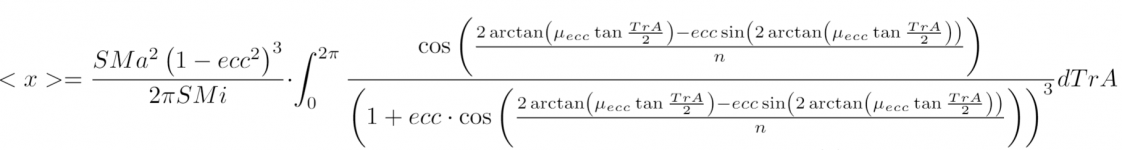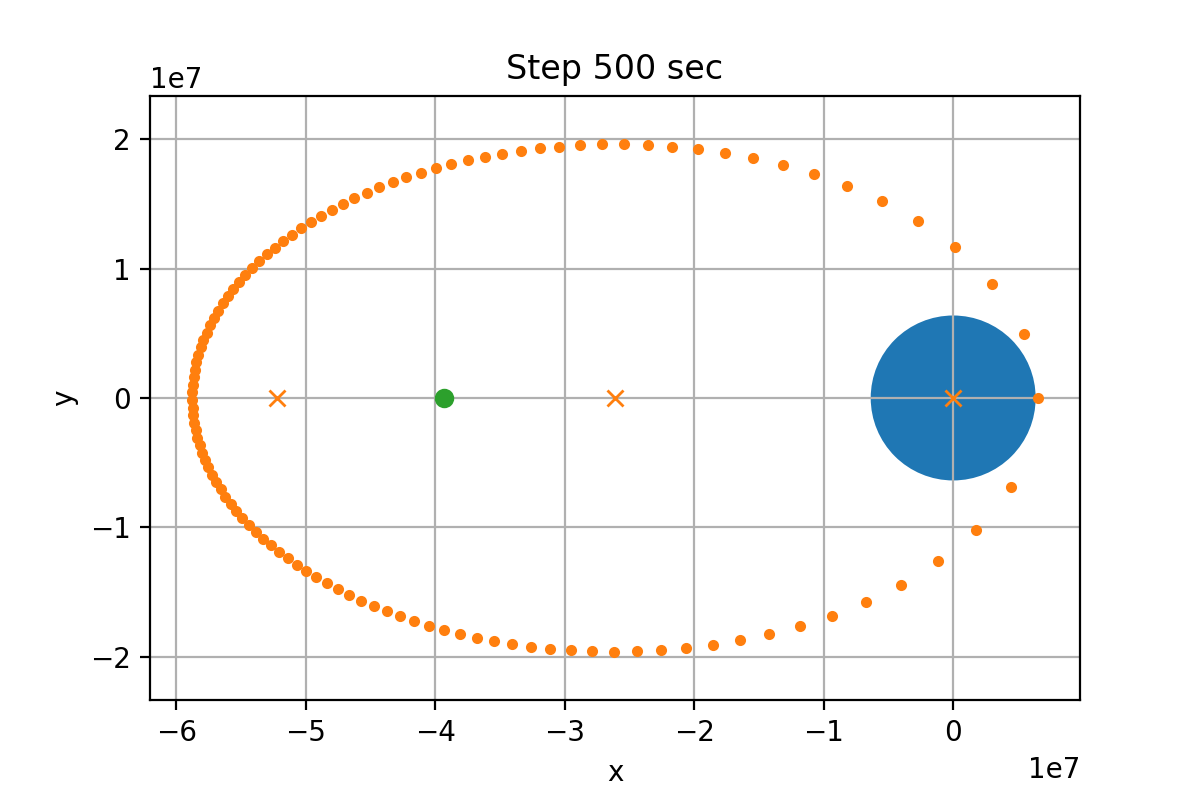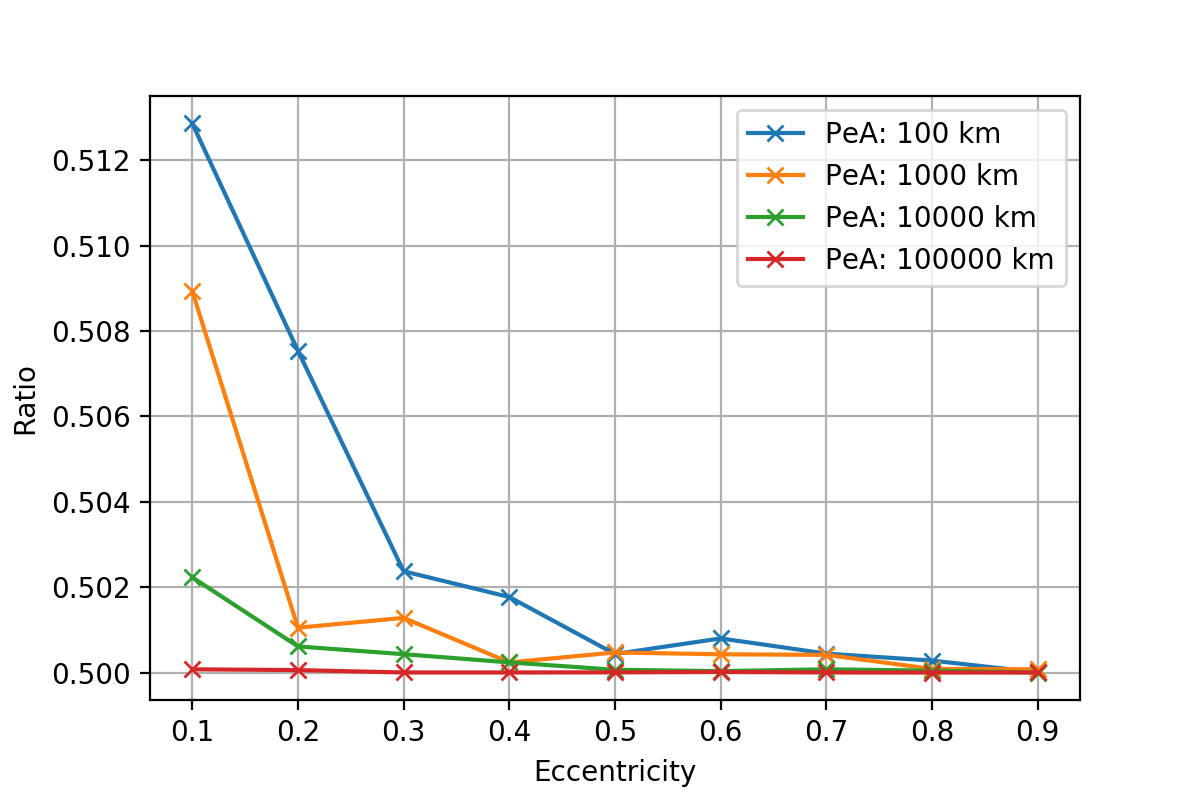# Average distance between objects on Keplerian orbits.

#### Linguofreak

##### Well-known member
Given a central body A, and two bodies B and C on Keplerian orbits around A, how easy is it to calculate the time-averaged distance between B and C?

For circular orbits it appears to be dead simple: the average position of both B and C is exactly the position of A, and the minimum possible distance is the difference of the two SMAs while the maximum possible distance is their sum, so the average distance should just be the SMA of the outermost orbit (I'm not sure there aren't some deviations from this if the two SMAs are nearly equal, but it seems to be a solid first approximation).

Can this be generalized to orbits with arbitrary elements (with the one constraint that eccentricity is strictly less than one) without making the math incredibly gnarly?

#### Thunder Chicken

Donator
Can this be generalized to orbits with arbitrary elements (with the one constraint that eccentricity is strictly less than one) ?

Seems do-able.

without making the math incredibly gnarly?

Ah, now we may have a problem...

#### C3PO

Donator
What about the plane(s)? A RInc other than zero would not help.

#### Linguofreak

##### Well-known member
What about the plane(s)? A RInc other than zero would not help.

Let's try the coplanar case, then the general case.

#### Linguofreak

##### Well-known member
In searching for resources on this, I found:

https://physicstoday.scitation.org/do/10.1063/PT.6.3.20190312a/full/

The point-circle method they describe is basically the logic I was using above to reason that the average distance between circular orbits should be roughly the radius of the outer orbit, though they worked out the math while I just guesstimated. As I suspected, the "radius of the outer orbit" rule works best when r_outer >> r_inner, but remains fairly accurate for orbital separations like those in the solar system.

EDIT: It also confirms the video RisingFury posted about Mercury being the closest on average.

---------- Post added at 12:00 ---------- Previous post was at 11:44 ----------

I guess one preliminary to developing an algorithm for the general case might be to figure out what the average position is for an object on an elliptical orbit. For the circular case, the average position is the center of the circle. For the elliptical case, I've got an intuition that says the average position is likely to be the empty focus of the ellipse, but I don't know how to calculate it rigorously to determine if that intuition is correct.

Last edited:

#### kuddel

##### Donator
Donator
"empty focus"? I am not familiar with this term, can you elaborate? (Just out of curiosity :thumbup#### Linguofreak

##### Well-known member
"empty focus"? I am not familiar with this term, can you elaborate? (Just out of curiosity :thumbupFor an elliptical orbit, the central body is at one focus of the ellipse. The other focus is empty.

#### kuddel

##### Donator
Donator
I assumed that, but wasn't sure I guessed right. Thanks for clarification.

And just by "guts feeling" I would say the average position of an ellipse might rather be the half-way point between the foci. either focus point is biased to "one side" of the ellipse...
But that's just my intuition, not real science#### kuddel

##### Donator
Donator
I think it is not very good to just look at one average position of an ellipse. Two objects in orbit are very affected by their "timing".
If their obits have the same period but are 180 "degrees apart" the "non-empty" focus might be the better point...

Again: Just rough thoughts here#### asbjos

##### tuanibrO
TL;DR: the average position is the midpoint between centre of ellipse and empty focus.

Prelude: I'm no mathematician. Expect errors. Get ready for many hand-wavy arguments. Some may be true, but some may be not.
I'm trying to find the average position of a satellite in an elliptical orbit, first analytically, then numerically.

Nomenclature:
y - vertical axis
x - horizontal axis
T - orbital period
TrA - true anomaly
MnA - mean anomaly
EcA - eccentric anomaly
n - mean motion
SMa - semi-major axis
SMi - semi-minor axis
ecc - eccentricity
μecc - an auxiliary number, defined as $$\mu_{ecc} = \sqrt{\frac{1-ecc}{1+ecc}}$$Animation from: https://commons.wikimedia.org/wiki/File:Elliptic_orbit.gif

Mathematical argument:
First, we agree to that the average position is somewhere on the axis through the two ellipse foci, and thus on the axis through the periapsis and apoapsis.
In mathematical language,
$$<y> = 0$$,
where the brackets denote the average/mean.
Then, the average x-position is the mean of all x positions in the orbit:
$$<x> = \frac{1}{T} \int^T_0 x(t) dt$$.
As the equation for an elliptical orbit is given in polar coordinates as
$$r(TrA) = \frac{SMa \left(1 - ecc^2\right)}{1+ecc \cos TrA}$$,
the x-position is
$$x(TrA) = r(TrA) \cdot \cos TrA$$.

Now, we must link the angle TrA to time t. The link will go through the mean anomaly MnA.
The mean anomaly is equal to the time from periapsis times the mean motion $$n = \frac{2 \pi}{T}$$, and the mean anomaly is from the Kepler Equation related to the eccentric anomaly, which is itself related to the true anomaly!
$$MnA = n \cdot t = EcA - ecc \cdot \sin EcA \Rightarrow t = \frac{EcA - ecc \cdot \sin EcA}{n}$$
We have that $$\tan \frac{EcA}{2} = \sqrt{ \frac{1-ecc}{1+ecc}} \tan \frac{TrA}{2} \Rightarrow EcA = 2 \arctan\left( \sqrt { \frac{1-ecc}{1+ecc}} \tan \frac{TrA}{2}\right)$$, so we then finally have a big equation to relate time t to the true anomaly TrA:
$$t = \frac{2 \arctan\left( \sqrt{\frac{1-ecc}{1+ecc}} \tan \frac{TrA}{2}\right) -ecc \sin \left(2 \arctan\left( \sqrt{\frac{1-ecc}{1+ecc}} \tan \frac{TrA}{2}\right)\right)}{n}$$.

Due to the LaTeX on Orbiter-Forum being limited to 400 characters (a limit I'll hit later), I'll have to define the number $$\mu_{ecc} = \sqrt{\frac{1-ecc}{1+ecc}}$$.

We must also convert the integral from dt to dTrA, which we can do from Kepler's second equation, which gives (look on Wikipedia if you don't believe me):
$$dt = r^2 \cdot \frac{T}{2\pi SMa SMi} dTrA$$.

We now get the original integral to become
$$<x> = \frac{1}{T} \int^T_0 x(t) dt = \frac{1}{T} \int^T_0 r(t) \cdot \cos(TrA(t)) dt = \frac{1}{2 \pi SMa SMi} \int^{2 \pi}_0 r(t)^3 \cdot \cos(TrA(t)) dTrA$$(uploaded as image, as forum doesn't support such a long equation).

This is where I stopped. I tried to let WolframAlpha and GeoGebra give it a go, but neither could. For all I know, I have done a mistake by now, so the equation may be wrong. But as you understand: finding an analytical answer is not the way for me to go.

Let's go to numerical "solving"!

Numerical argument:
Much simpler for me, especially considering my recent programming efforts with orbital calculations.

Using Python, I do much the same as what I attempted to generalise above: put an object in orbit with some semi-major axis and eccentricity, and then calculate the position every second (or other interval), and find the average.

Fairly easy, and we get the figure below:Here we see the position of the satellite every 500 seconds, from the perigee at 160 km, with eccentricity 0.800. The three crosses from the left to right are the empty focus (x=PeR-ApR in graph coordinates), centre of the ellipse (x=PeR-SMa in graph coordinates), and the focus with the Earth's centre (x=0 in graph coordinates).
The average position is the green dot.
Wait a second, doesn't that seem to be in the middle between the left focus and the centre of the ellipse?
Calculating the ratio between (average position - centre of ellipse) and (left focus - centre of ellipse), we find that value to be 0.5067.

Reducing the step size to 10 seconds, and checking elliptical orbits with eccentricity 0.1 -- 0.9 and PeA 100 km, 1 000 km, 10 000 km and 100 000 km, we get the following ratios:As you can see, when increasing steps (i.e. longer period, either by higher perigee or eccentricity), we get values closer and closer to 1/2.

So using this "evidence", we can quite safely say that the average position of an object in a Keplerian orbit is the midpoint between the empty focus and ellipse centre, or precisely $$<x> = PeR - 0.5 \cdot SMa - 0.5 \cdot ApR = SMa \cdot \left(1.5 ecc\right)$$ in our coordinate system, which is distance $$1.5 \cdot SMa \cdot ecc$$ from the planet centre in the direction of the apoapsis for any elliptical orbit.

Coda:
I assume the average distance between two independent satellites is the distance between their average positions (the point I've found above). As is mentioned by kuddel, this is not true for objects with equal periods, and maybe also not for objects with rational period fractions. But for every irrational period fraction, I assume this mean-point-to-mean-point distance to be the average distance when we let the time pass.

EDIT 25.09.2020: changed from old forum $-$ to $$-$$ formatting.

Last edited:

#### Linguofreak

##### Well-known member
So using this "evidence", we can quite safely say that the average position of an object in a Keplerian orbit is the midpoint between the empty focus and ellipse centre, or precisely $<x> = PeR - 0.5 \cdot SMa - 0.5 \cdot ApR = SMa \cdot \left(1.5 ecc\right)$ in our coordinate system, which is distance $1.5 \cdot SMa \cdot ecc$ from the planet centre in the direction of the apoapsis for any elliptical orbit.

Another way of looking at it is that it is at distance $SMa \cdot \left(0.5 ecc\right)$ from the empty focus in the direction of the planet.

Coda:
I assume the average distance between two independent satellites is the distance between their average positions (the point I've found above). As is mentioned by kuddel, this is not true for objects with equal periods, and maybe also not for objects with rational period fractions. But for every irrational period fraction, I assume this mean-point-to-mean-point distance to be the average distance when we let the time pass.

Not quite. In the circular, coplanar case, this would predict an average distance of zero, regardless of period ratios. In that case, taking the average distance of the outer object from the average position of the inner object works (which works out, for that case, to just the SMa of the outer orbit). I have no idea if that generalizes.

#### kuddel

##### Donator
Donator
My thoughts on this:
When you consider the "time spent" over the obit, the average-center-position is clearly more shifted towards the apoapsis side, as it stays longer there than on the periapsis side.

I would assume, that if you plot the two obits as a "distance to central body" on the same graph you'll get two sinusodial plots. If you then add them up (or rather subtract them; depends on you zero line), you get another sinusodial plot of which you can get the average value as an average distance.

To better show what I mean I've added an image. In that image, red and blue are the bodies distances, the dashed green is their "sum",
The average of that sum (not draw in the image) might be a good value..

Last edited:

#### Linguofreak

##### Well-known member
Not quite. In the circular, coplanar case, this would predict an average distance of zero, regardless of period ratios. In that case, taking the average distance of the outer object from the average position of the inner object works (which works out, for that case, to just the SMa of the outer orbit). I have no idea if that generalizes.

Coming back to this, assuming

1) An object O on an arbitrary Keplerian orbit around the origin of the coordinate system in use.
2) An arbitrary point P in 3D space.

How complicated is it to calculate the time-averaged distance between O and P?